Reach Us+44-1474-556909
Calculation of Value of andpi; by Splitting Technique (2013) | OMICS International
Journal of Applied & Computational Mathematics
All submissions of the EM system will be redirected to Online Manuscript Submission System. Authors are requested to submit articles directly to Online Manuscript Submission System of respective journal.

# Calculation of Value of π by Splitting Technique (2013)

Fazal Rehman Abasyeen Khan* and Qadeem Khan

Kohat University of Science and Technology (K.P.K) Pakistan

*Corresponding Author:
Fazal Rehman Abasyeen Khan
Kohat University of Science and Technology (K.P.K) Pakistan
Tel: 0922-5291501-502
E-mail: [email protected]

Received Date: October 17, 2014; Accepted Date: January 23, 2015; Published Date: January 30, 2015

Citation: Khan FRA, Khan Q (2015) Calculation of Value of π by Splitting Technique (2013). J Appl Computat Math 4:200. doi: 10.4172/2168-9679.1000200

Copyright: © 2015 Khan FRA, et al. This is an open-access article distributed under the terms of the Creative Commons Attribution License, which permits unrestricted use, distribution, and reproduction in any medium, provided the original author and source are credited.

Visit for more related articles at Journal of Applied & Computational Mathematics

#### Abstract

This paper proposes a geometrical technique to obtain the value of π from splitting a circle in Equilateral triangles, a Square and Sectors. It is first ever study to calculate the value of π by splitting a circle in these shapes and then by combining areas of these shapes value of π can be calculated.

#### Introduction

The quest for π has a long history . The fact that the ratio of circle’s circumference to its diameter is a constant has long been known. The ratio first enters human consciousness in Egypt. The Egyptian Rhind Papyrus, dating back to 1650 B.C, provides the good evidence that π equals 4(8/9)2 ≈ 3.16. In the Bible, there are also records of the value ofπ . The biblical value2 of π is 3. In ancient Greece, the first approximation of π was obtain by Archimedes (287-212 B.C.), who showed that 223/7 <π < 22/7. In ancient China, the calculation of π was documented in the 1000 B.C. In Zhoung Bi Suan Jing, one of the oldest Chinese astronomical and mathematical treatises. It states that the circumference of a circle is about 3 times its diameter. Heng Zhang (78-139) approximated π by 92/299 (≈3.174) and √10 (=3.1622). In around 263, Hui Lui calculated π to be 3.141024. Zu3 (429-500) showed that the value of π lies between 3.1415926 and 3.1415927 [1,2]. Since Zu, the calculation of π made little progress for almost a millennium. During the past five centuries, the following attempts have been made to obtain the value ofπ .

The notation π for the circumference-to-diameter ratio was first introduced in 1706 by William jones (1675-1749) in his book “A new introduction to Mathematics; and was made by popular by Leonard Euler (1707-1783). The number has remained as π ever since.

A verse of the Bible reads: And he made a molten sea, ten cubits from the one brim to the other: it was round all about, and his height was five cubits: and a line of thirty cubits did compass it about  (1 kings 7, 23).

Chongzhi Zu was the first to obtain the formula for the volume of a sphere. He developed the best calendar known to people at that time and was the first to calculate the time needed for the Earth to orbit the Sun as 365.24281481 days, which is correct up to 3 decimal places. Today, a volcano on the moon is named after Zu in recognition of his contributions .

Francois Viète (1540-1603), a French mathematician .

Johns Wallis (1616-1703), a British mathematician .

π Was shown to be an irrational in 1767 by Lambert . It was proved to be a transcendental number in 1882 by Linderman . Buffon  was the first to obtain π via random experiment . Since Buffon, more results in probability theory related to π have been found [10-15] with the invention of computing machines, more precise values of π could be calculated. In1949, the US army obtained the value of π up to 2035 decimal places. Borwein and Borwein  proposed the following algorithm for the calculation of π up to billion places:

One can show that 1/α3 is very close to π and 1/ α15 is identical to π for more than two billion decimals [16-19]. In 1994, the Chdnovsky brothers obtained the value of π up to 4 billion decimal places and Kanada and Takashi calculated π up to 206 billion decimal places in 1999. For more information on the computation of π , one is referred to . In this paper a new method to obtain the value of π is proposed . The method is based on splitting a circle in a square, equilateral triangles and Sectors. We find good evidence that π can be obtained by combining areas of above mention shapes inside given circle (Figure 1).

#### Steps of Construction

(I) Draw a circle of radius R with centre O.

(ii) Draw another circle having radius r =R/2 with centre O.

(iii) Make angles of 0º, 45º, 90º, 135º, 180º, 225º, 270º, and 315º inside given circle.

(iv) Joining end points of angles 0º and 180º.

(v) Joining end points of angles 45º and 225º.

(vi) Joining end points of angles 90º and 270º.

(vi) Joining end points of angles 135º and 315º.

(vii) Joining A and C, B and D, and so on.

(vii) It is required split circle.

#### Obtaining from Splitting A Circle

In this technique a given circle having radius R and Centre is O split into a square, four equilateral triangles and then by adding areas of these geometrical shapes inside given circle value of can be calculated easily and efficiently.

Let

Measurement of each side of square=x units

Measurement of each side of equilateral triangle=x units

Measurement of radius of each sector=x units

Area of circle = 4(Area of Equilateral Triangle) + (Area of square) + 4(Area of sector)……………      (1)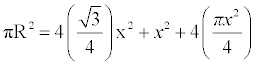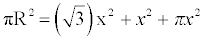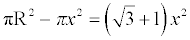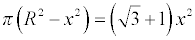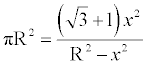(2)

R=IO+AI      (3)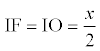(4)

AF=GH=EG=EF=x      (5)

AIF is right angled triangle

AI=?      (6)

By Applying Pythagoras theorem value of AI can be calculated as.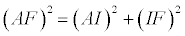(7)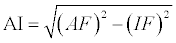(8)

By putting value of AF and IF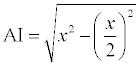(9)

By putting value of IO and AI in (iii) we get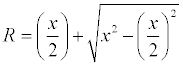(10)

Now putting value of R in (ii)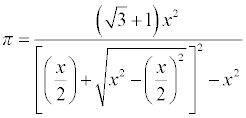(11)

It is general formula of π

4. Solving general form new formula

Now By solving (XI) we get value of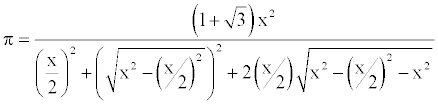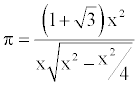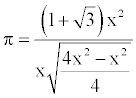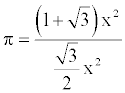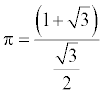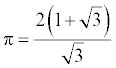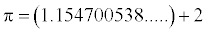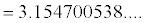It is value of π

6. Calculating value of π while using 9 as arbitrarily number

Put x = 9 cm in (xi) we have Put x = 9 cm in (xi) we have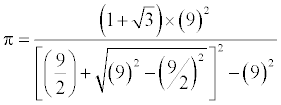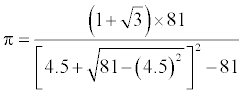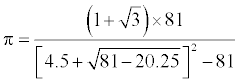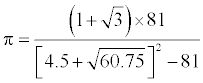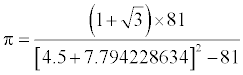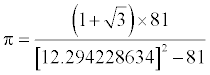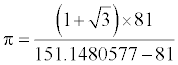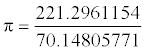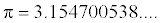#### References

Select your language of interest to view the total content in your interested language

### Article Usage

• Total views: 11919
• [From(publication date):
February-2015 - Jul 16, 2019]
• Breakdown by view type
• HTML page views : 8145Can't read the image? click here to refresh